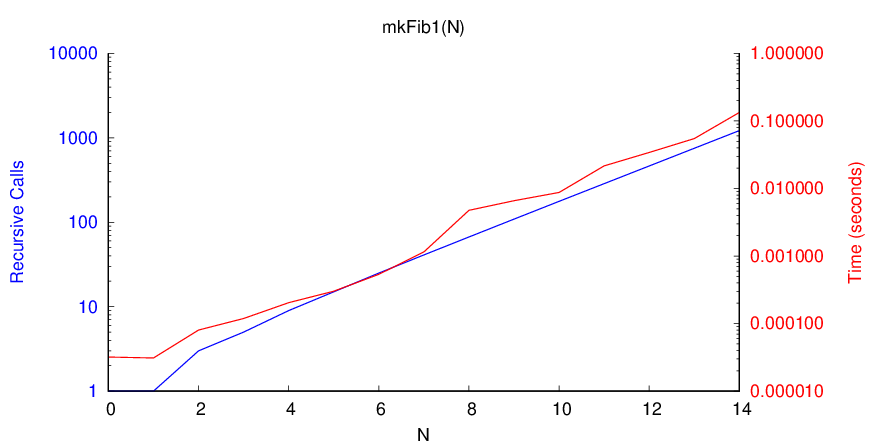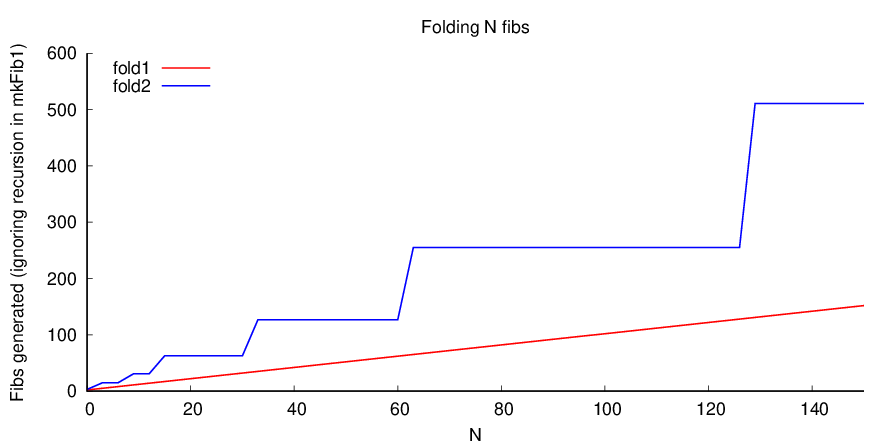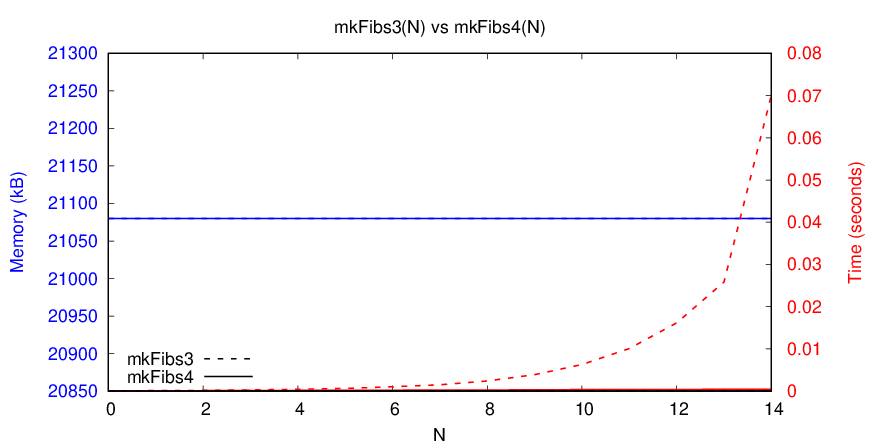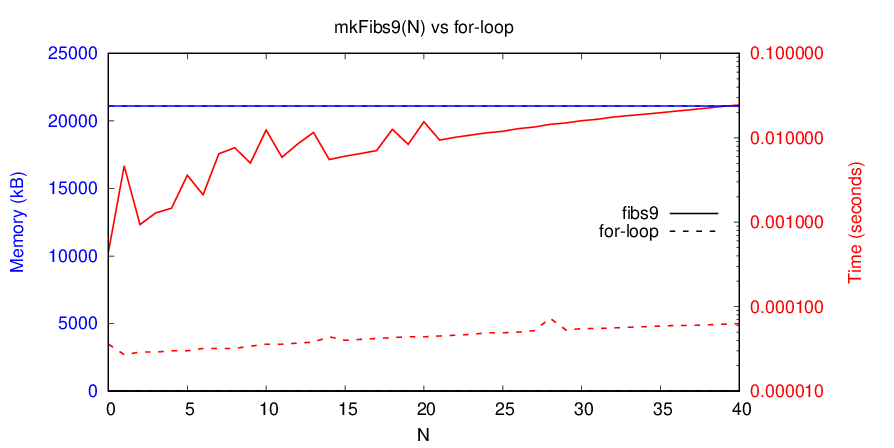# Fibonacci Sequence in PHP

Posted on by Chris Warburton

## Exponential Definitions

The Fibonacci sequence is a well-known series of numbers which follow a pattern: it starts `1, 1` and each subsequent number is the sum of the two preceding it. We can calculate the `\$n`th element of the sequence like this in PHP (using handy functions from PHP Prelude):

``````defun('mkFib1', function(\$n) {
return (\$n < 2)? 1  // Base case
: mkFib1(\$n - 1) + mkFib1(\$n - 2);
});``````

This is an incredibly naive approach, since each call to `mkFib1` can involve 2 more calls to `mkFib1`. This recursion is well-founded, since it will always head towards the base case where `\$n < 2`, but there are two problems:

• It’s not tail-recursive, so it requires a linear number of stack frames, leading to stack overflows.
• It requires an exponential number of recursive calls to reach a base case, which gives us an exponential running time:What’s more, a function is a rather clumsy way of representing a sequence, since the structure isn’t immediately available and must be inferred by our users via some sort of counter. This violates the Once and Only Once rule, and is liable to cause off-by-one errors and such.

So what’s the alternative? The trick is to realise that we don’t need to provide every element, since our users will only use a finite number. The difficulty is that we don’t know how many they’ll need!

One simple approach that may spring to mind is asking the user up front how many elements they need:

``defun('mkFibs2', compose(map('mkFib1'), 'upto'));``

However, this doesn’t do what we want. The requirement to know how many elements are needed is too strong; many useful functions like filter and fold (AKA “reduce”) require an unknown number of elements in general; for example, filters don’t know how many elements they’ll need to discard before finding a match. This forces us to generate longer and longer sequences, slowing us down by a logarithmic factor compared to `mkFib1`:

``````// Note: Since there are infinitely many fibs, we can't fold all of them.
//       Instead, \$f returns a pair [\$stop, \$result], where \$stop tells us
//       when to cut-off the fold and \$result is treated in the usual way.

// Fold a sequence generated by mkFib1
defun('fold1', function(\$f) {
// Fold over the Naturals \$n = 0, 1, 2, ...
return loop(function(\$x, \$n) use (\$f) {
return \$f(\$x, mkFib1(\$n));
});
});

// Prevents us applying \$f to too many fibs
defun('shortcircuit', function(\$f, \$x, \$fib) {
return \$x? \$x  // If \$x says stop, return it as-is
: \$f(\$x, \$fib);  // Else call \$f
});

// Fold a sequence generated by mkFib2
defun('fold2', function(\$f) {
// Fold over the Naturals \$n = 0, 1, 2, ...
return loop(function(\$x, \$n) use (\$f) {
// Fold over 2^\$n fibs
list(\$s, \$y) = array_reduce(mkFibs2(pow(2, \$n)),
shortcircuit(\$f),
[false, \$x]);
return [\$s, \$s? \$y    // If \$s(top), return \$y
});
});``````In this blog post I’ll show how we can improve all these things, ending up with a structured representation that, for any unknown N, can fold N elements in O(1) memory, O(N) time and O(1) stack frames.

## Inductive Definitions

The problem with `mkFib2` is that the size of a PHP array must be specified in advance, but we don’t know how many elements we’ll need. One simple alternative to the array is a list. Rather than storing all elements in a single place, a list stores them separately, then links them all together. There are many different kinds of list, but the simplest is called tail recursive (or “singly-linked”) and can be implemented very easily in PHP by nesting arrays:

``````// \$x is our current element, \$n is when to stop
defun('mkFibs3_', function(\$x, \$n) {
return (\$x < \$n)? [mkFib1(\$x), mkFibs3_(\$x+1, \$n)]
: [];
});
defun('mkFibs3',  mkFibs3_(0));  // Always start at 0``````

The results look like this:

``````
array(2) {
=>
int(1)
=>
array(2) {
=>
int(1)
=>
array(2) {
=>
int(2)
=>
array(2) {
=>
int(3)
=>
array(2) {
=>
int(5)
=>
array(0) {
}
}
}
}
}
}
``````

The results of `mkFibs3` still have a finite size, but we’re no longer at the mercy of PHP’s implementation details. Each array has a known size (`0` or `2`), no matter how many elements we ask for. Like `mkFib1`, `mkFibs3` isn’t tail recursive, so it may overflow the stack if we ask for too many elements. Folding lists is a little awkward in PHP, since there’s no built-in equivalent to `array_reduce`:

``````defun('fold3', function(\$f, \$x) {
return trampoline(y(
function(\$g, \$n, \$fibs, \$acc, \$_) use (\$f, \$x) {
// If we've run out of \$fibs, start again with more
if (\$fibs === [])
return [false, \$g(\$n+1, mkFibs3(pow(2, \$n+1)), \$x)];

// Apply \$f to the next \$fib
list(\$stop, \$acc) = \$f(\$acc, \$fibs);
return [\$stop, \$stop? \$acc
: \$g(\$n, \$fibs, \$acc)];
}, 0, [], null));
});``````

We can now do away with the exponentially-inefficient `mkFib1`. If we log the arguments we’re sending to `mkFib1`, we can see that `mkFibs3` is asking for the same values over and over:

mkFibs3(…) mkFib1(…)
0
1 0
2 0, 1
3 0, 1, 2, 1, 0
4 0, 1, 2, 1, 0, 3, 2, 1, 0, 1
5 0, 1, 2, 1, 0, 3, 2, 1, 0, 1, 4, 3, 2, 1, 0, 1, 2, 1, 0
6 0, 1, 2, 1, 0, 3, 2, 1, 0, 1, 4, 3, 2, 1, 0, 1, 2, 1, 0, 5, 4, 3, 2, 1, 0, 1, 2, 1, 0, 3, 2, 1, 0, 1

We can actually remove all of these calls, since `mkFibs3` has all of the data it needs to construct each Fibonacci number itself. If we pass this data along the recursive calls, our runtime becomes linear:

``````defun('mkFibs4_', function(\$fib_2, \$fib_1, \$x, \$n) {
if (\$x >= \$n) return [];  // Stopping condition
\$fib = (\$x < 2)? 1 : (\$fib_2 + \$fib_1);
return [\$fib, mkFibs4_(\$fib_1, \$fib, \$x+1, \$n)];
});
defun('mkFibs4',  mkFibs4_(null, null, 0));``````## Coinductive Definitions

Now we can deal with the finiteness problem. Every time PHP sees one of our lists, it does the following:

• Construct the first element (the Fibonacci number, known as the car or head)
• Construct the second element (the rest of the list, known as the cdr or tail)
• Construct the array containing them

Our problem is that we’re forced to construct the whole tail before we know how long it needs to be. The solution is to delay the tail, which we can do using a thunk: a function which returns a constant value. PHP will construct functions without running them, which gives us time to figure out how many elements we need.

``````defun('mkFibs5_', function(\$fib_2, \$fib_1, \$x, \$n) {
if (\$x >= \$n) return [];  // Stopping condition
\$fib = (\$x < 2)? 1 : (\$fib_2 + \$fib_1);
return [\$fib, function(\$_) use (\$fib_1, \$fib, \$x, \$n) {
return mkFibs5_(\$fib_1, \$fib, \$x+1, \$n);
}];
});
defun('mkFibs5',  mkFibs5_(null, null, 0));``````

Delaying computation this way is known as lazy evaluation, and this method of generating a bit of data and delaying the rest is called co-induction. Hence this kind of structure is known as a co-data structure.

The nice thing about codata is that we can define never-ending chains of values, wrapping each link in a thunk so that it only gets evaluated when needed (known as forcing the value). This is exactly what we need for our Fibonacci sequence, and all we need to do is throw away the stopping condition!

Notice that I’m giving these thunks a parameter `\$_` which is ignored. As far as PHP’s concerned, I could have defined them as nullary functions, ie. taking no arguments, but that makes them harder to reason about and prevents some later simplifications:

``````defun('fibsFrom6', function(\$fib_2, \$fib_1) {
\$fib = \$fib_2 + \$fib_1;
return [\$fib, function(\$_) use (\$fib_1, \$fib) {
return fibsFrom6(\$fib_1, \$fib);
}];
});
defun('fibs6',     function(\$_) {
return [1, function(\$_) {
return [1, function(\$_) {
return fibsFrom6(1, 1);
}];
}];
});``````

This is the first of our definitions that’s both infinite, like a function, and structured, like an array. This kind of codata structure is called a stream. Here’s how to fold a stream, using a trampoline to optimise tail-calls:

``````defun('fold6',  function(\$f, \$acc, \$stream) {
return trampoline(y(function(\$y, \$acc, \$s, \$_) use (\$f) {
list(\$h,    \$t)    = \$s(null);
list(\$stop, \$acc)  = \$f(\$acc, \$h);
return [\$stop, \$stop? \$acc
: \$y(\$acc, \$t)];
}, \$acc, \$stream));
});``````

### Refactoring

Now that we’ve created our infinite Fibonacci sequence, we can refactor the code to be a little less naff. I debated whether to define the `fibsFrom` function inline, but I think it’s nice to keep two separate functions since they correspond exactly to the two rules defining the Fibonacci sequence: `fibsFrom` implements “sum the previous two”, `fibs` implements “start with `1`, `1`”.

The first refactoring we can do is to notice that we have functions returning functions, which is a manual form of currying. We can remove this separation, since our functions are curried automatically by `defun` (we couldn’t do this if our thunks were nullary):

``````defun('fibsFrom7', function(\$l, \$m, \$_) {
\$n = \$l + \$m;
return [\$n, fibsFrom7(\$m, \$n)];
});
defun('fibs7',     function(\$_) {
return [1, function(\$_) {
return [1, fibsFrom7(1, 1)];
}];
});``````

Next we can remove the redundant `1`s in `fibs7`. This redundancy is due to `fibsFrom` not including its arguments in the stream it returns, but of course if we naively change `fibsFrom` to include its arguments, we’d just be shifting around the redundancy, not eliminating it.

The solution is to extrapolate the sequence backwards a couple of steps. In other words, we need to switch the initial `1, 1` to some other values `\$x` and `\$y` such that we get a sequence `\$x, \$y, 1, 1, 2, 3, 5, 8, ...`. We can derive these values straight from the definition of the Fibonacci sequence:

``````\$y + 1 == 1      // Since \$y, 1, 1 is in the sequence
\$y     == 1 - 1
\$y     == 0

\$x + \$y == 1  // Since \$x, \$y, 1 is in the sequence
\$x + 0  == 1  // From the value of \$y above
\$x      == 1``````

Now we can pass `\$x` and `\$y` as arguments to `fibsFrom` and get back a stream of all fibs after `\$x` and `\$y`, which is what we want. This simplifies our definition considerably:

``defun('fibs8', fibsFrom7(1, 0));``

Finally, we can collapse `fibsFrom` into a one-liner, since PHP evaluates array elements in-order:

``````defun('fibsFrom9', function(\$l, \$m, \$_) {
return [\$n = \$l + \$m, fibsFrom9(\$m, \$n)];
});
defun('fibs9',     fibsFrom9(1, 0));``````

### Results

This is quite elegant, so let’s leave it there and see how well our fold performs. Note that we can reuse `fold6`, since our interface hasn’t changed:Note that the memory usage is constant, and it turns out we don’t pay any memory penalty for using streams compared to a hard-coded for-loop. We do pay a time penalty, most likely from all of the function calls involved, but it’s only a constant factor so, as the log scale shows, the scaling behaviour isn’t affected.

So there we have it, a PHP implementation of the Fibonacci sequence which:

• Has a sequential structure, like the sequence itself
• Is never-ending, like the sequence itself (thanks to co-induction)
• Uses constant stack-space (thanks to tail-call optimisation, manually implemented with trampolines)
• Uses constant memory (thanks to lexical closures)
• Uses linear time (again, thanks to lexical closures)
• Closely follows the two defining rules of the sequence
• Has a self-contained, abstract, composable, functional interface
• Only requires 4 lines to define, not counting the generic library functions from `prelude.php`
``````defun('fibsFrom', function(\$l, \$m, \$_) {
return [\$n = \$l + \$m, fibsFrom(\$m, \$n)];
});
defun('fibs',     fibsFrom(1, 0));``````